Updating search results...

# 13 Results

View
Selected filters:
• MCCRS.Math.Content.7.NS.A.2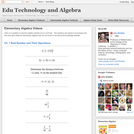Conditional Remix & Share Permitted
CC BY-NC
Rating
0.0 stars

Visually searchable database of Algebra 1 videos. Click on a problem and watch the solution on YouTube. Copy and paste this material into your CMS. Videos accompany the open Elementary Algebra textbook published by Flat World Knowledge.

Subject:
Algebra
Mathematics
Material Type:
Lecture
Lecture Notes
Provider:
Individual Authors
Provider Set:
Individual Authors
Author:
John Redden
04/29/2012Educational Use
Rating
0.0 stars

Students do work by lifting a known mass over a period of time. The mass and measured distance and time is used to calculate force, work, energy and power in metric units. The students' power is then compared to horse power and the power required to light 60-watt light bulbs.

Subject:
Applied Science
Engineering
Physical Science
Physics
Material Type:
Activity/Lab
Provider:
TeachEngineering
Provider Set:
TeachEngineering
Author:
Jan DeWaters
Susan Powers
09/18/2014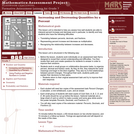Only Sharing Permitted
CC BY-NC-ND
Rating
0.0 stars

This lesson unit is intended to help teachers assess how well students are able to interpret percent increase and decrease, and in particular, to identify and help students who have the following difficulties: translating between percents, decimals, and fractions; representing percent increase and decrease as multiplication; and recognizing the relationship between increases and decreases.

Subject:
Mathematics
Ratios and Proportions
Material Type:
Assessment
Lesson Plan
Provider:
Shell Center for Mathematical Education
Provider Set:
Mathematics Assessment Project (MAP)
04/26/2013Conditional Remix & Share Permitted
CC BY-NC
Rating
0.0 stars

Four full-year digital course, built from the ground up and fully-aligned to the Common Core State Standards, for 7th grade Mathematics. Created using research-based approaches to teaching and learning, the Open Access Common Core Course for Mathematics&nbsp;is designed with student-centered learning in mind, including activities for students to develop valuable 21st century skills and academic mindset.

Subject:
Mathematics
Material Type:
Full Course
Provider:
Pearson
10/06/2016Conditional Remix & Share Permitted
CC BY-NC
Rating
0.0 stars

Working With Rational Numbers

Type of Unit: Concept

Prior Knowledge

Students should be able to:

Compare and order positive and negative numbers and place them on a number line.
Understand the concepts of opposites absolute value.

Lesson Flow

The unit begins with students using a balloon model to informally explore adding and subtracting integers. With the model, adding or removing heat represents adding or subtracting positive integers, and adding or removing weight represents adding or subtracting negative integers.

Students then move from the balloon model to a number line model for adding and subtracting integers, eventually extending the addition and subtraction rules from integers to all rational numbers. Number lines and multiplication patterns are used to find products of rational numbers. The relationship between multiplication and division is used to understand how to divide rational numbers. Properties of addition are briefly reviewed, then used to prove rules for addition, subtraction, multiplication, and division.

This unit includes problems with real-world contexts, formative assessment lessons, and Gallery problems.

Subject:
Algebra
Mathematics
Material Type:
Unit of Study
Provider:
Pearson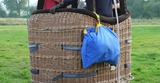Conditional Remix & Share Permitted
CC BY-NC
Rating
0.0 stars

Students use number lines to represent products of a negative integer and a positive integer, and they use patterns to understand products of two negative integers. Students write rules for products of integers.Key ConceptsThe product of a negative integer and a positive integer can be interpreted as repeated addition. For example, 4 &bull; (&ndash;2) = (&ndash;2) + (&ndash;2) + (&ndash;2) + (&ndash;2). On a number line, this can be represented as four arrows of length 2 in a row, starting at 0 and pointing in the negative direction. The last arrow ends at &ndash;8, indicating that 4 &bull; (&ndash;2) = &ndash;8. In general, the product of a negative integer and a positive integer is negative.The product of two negative integers is hard to interpret or visualize. In this lesson, we use patterns to help students see why a negative integer multiplied by a negative integer equals a positive integer. For example, students can compute the products in the pattern below.4 &bull; (&ndash;3) = &ndash;123 &bull; (&ndash;3) = &ndash;92 &bull; (&ndash;3) = &ndash;61 &bull; (&ndash;3) = &ndash;30 &bull; (&ndash;3) = 0They can observe that, as the first factor decreases by 1, the product increases by 3. They can continue this pattern to find these products.&ndash;1 &bull; (&ndash;3) = 3&ndash;2 &bull; (&ndash;3) = 6&ndash;3 &bull; (&ndash;3) = 9In the next lesson, we will prove that the rules for multiplying positive and negative integers extend to all rational numbers, including fractions and decimals.Goals and Learning ObjectivesRepresent multiplication of a negative integer and a positive integer on a number line.Use patterns to understand products of two negative integers.Write rules for multiplying integers.

Subject:
Numbers and Operations
Material Type:
Lesson Plan
09/21/2015Conditional Remix & Share Permitted
CC BY-NC
Rating
0.0 stars

Students solve division problems by changing them into multiplication problems. They then use the relationship between multiplication and division to determine the sign when dividing positive and negative numbers in general.Key ConceptsThe rules for determining the sign of a quotient are the same as those for a product: If the two numbers have the same sign, the quotient is positive; if they have different signs, the quotient is negative. This can be seen by rewriting a division problem as a multiplication of the inverse.For example, consider the division problem &minus;27 &divide; 9. Here are two ways to use multiplication to determine the sign of the quotient:The quotient is the value of x in the multiplication problem 9 &sdot; x = &minus;27. Because 9 is positive, the value of x must be negative in order to get the negative product.The division &minus;27 &divide; 9 is equivalent to the multiplication &minus;27 &sdot; 19. Because this is the product of a negative number and a positive number, the result must be negative.Goals and Learning ObjectivesUse the relationship between multiplication and division to solve division problems involving positive and negative numbers.Understand how to determine whether a quotient will be positive or negative.

Subject:
Numbers and Operations
Material Type:
Lesson Plan
09/21/2015Conditional Remix & Share Permitted
CC BY-NC
Rating
0.0 stars

Students use properties of multiplication to prove that the product of any two negative numbers is positive and the product of a positive number and a negative number is negative.Key ConceptsMultiplication properties can be used to develop the rules for multiplying positive and negative numbers.Students are familiar with the properties from earlier grades:Associative property of multiplication: Changing the grouping of factors does not change the product. For any numbers a, b, and c, (a &sdot; b) &sdot; c = a &sdot; (b &sdot; c).Commutative property of multiplication: Changing the order of factors does not change the product. For any numbers a and b, a &sdot; b = b &sdot; a.Multiplicative identity property of 1: The product of 1 and any number is that number. For any number a, a &sdot; 1 = 1 &sdot; a = a.Property of multiplication by 0: The product of 0 and any number is 0. For any number a, a &sdot; 0 = 0 &sdot; a = 0.Property of multiplication by &minus;1: The product of &minus;1 and a number is the opposite of that number. For any number a, (&minus;1) &sdot; a = &minus;a.Existence of multiplicative inverses: Dividing any number by the same number equals 1. Multiplying any number by its multiplicative inverse equals 1. For every number a &ne; 0, a &divide; a = a &sdot; 1a = 1a &sdot; a = 1.Distributive property: Multiplying a number by a sum is the same as multiplying the number by each term and then adding the products. For any numbers a, b, and c, a &sdot; (b + c) = a &sdot; b + a &sdot; c.In this lesson, students will encounter a proof showing that the product of a positive number and a negative number is negative and two different proofs that the product of two negative numbers is positive. Two alternate proofs are as follows.Proof that the product of two negative numbers is positive:Represent the negative numbers as &minus;a and &minus;b, where a and b are positive.(&minus;a) &sdot; (&minus;b)Original expression= ((&minus;1) &sdot; a) &sdot; ((&minus;1) &sdot; b)&nbsp;&nbsp; Property of multiplication by &minus;1= (&minus;1) &sdot; (a &sdot; (&minus;1)) &sdot; b&nbsp;&nbsp; Associative property of multiplication= (&minus;1) &sdot; ((&minus;1) &sdot; a) &sdot; b&nbsp;&nbsp; Commutative property of multiplication= ((&minus;1) &sdot; (&minus;1)) &sdot; (a &sdot; b)&nbsp;&nbsp; Associative property of multiplication= 1 &sdot; (a &sdot; b)&nbsp;&nbsp; Property of multiplication by &minus;1= a &sdot; b&nbsp;&nbsp; Multiplicative identity property of 1Because a and b are positive, a &sdot; b is positive.Proof that the product of a positive number and a negative number is negative:Let a be the positive number. Let &minus;b be the negative number, where b is positive.a &sdot; (&minus;b)Original expression= a &sdot; ((&minus;1) &sdot; b)&nbsp;&nbsp;&nbsp;&nbsp; Property of multiplication by &minus;1= (a &sdot; (&minus;1)) &sdot; b&nbsp;&nbsp;&nbsp;&nbsp; Associative property of multiplication= ((&minus;1) &sdot; a) &sdot; b&nbsp;&nbsp;&nbsp;&nbsp; Commutative property of multiplication= (&minus;1) &sdot; (a &sdot; b)&nbsp;&nbsp;&nbsp;&nbsp; Associative property of multiplication= &minus;(a &sdot; b)&nbsp;&nbsp;&nbsp;&nbsp; Property of multiplication by &minus;1Because a and b are positive, a &sdot; b is positive, so &minus;(a &sdot; b) must be negative.Goals and Learning ObjectivesReview properties of multiplication.Explain why the product of two negative numbers is positive and the product of a negative number and a positive number is negative.

Subject:
Numbers and Operations
Material Type:
Lesson Plan
09/21/2015Conditional Remix & Share Permitted
CC BY-NC
Rating
0.0 stars

Students critique and improve their work on the Self Check. They then extend their knowledge with additional problems.Students solve problems that require them to apply their knowledge of multiplying and dividing positive and negative numbers. Students will then take a quiz.Key ConceptsTo solve the problems in the Self Check, students must apply their knowledge of multiplication and division of positive and negative numbers learned throughout the unit.Goals and Learning ObjectivesUse knowledge of multiplication and division of positive and negative numbers to solve problems.

Subject:
Numbers and Operations
Material Type:
Lesson Plan
09/21/2015Conditional Remix & Share Permitted
CC BY-NC
Rating
0.0 stars

Students use the distributive property to rewrite and solve multiplication problems. Then they apply addition and multiplication properties to simplify numerical expressions.Key ConceptsThe distributive property is stated in terms of addition: a(b + c) = ab + ac, for all numbers a, b, and c. However, it can be extended to subtraction as well: a(b &minus; c) = ab &minus; ac, for all numbers a, b, and c. Here is a proof. (We have combined some steps.)a(b &minus; c)Original expression= a(b + (&minus;c))Subtracting is adding the opposite.= a(b) + a(&minus;c)Apply the distributive property.= ab + a(&minus;1 &sdot; c)Apply the property of multiplication by &minus;1.= ab + &minus;1(ac)Apply the associative and commutative properties of multiplication.= ab + &minus;(ac)Apply the property of multiplication by &minus;1.= ab &minus; acAdd the opposite is subtracting.We can use the distributive property to make some multiplication problems easier to solve. For example, by rewriting \$1.85 as \$2.00 &minus; \$0.15 and applying the distributive property, we can change 6(\$1.85) to a problem that is easy to solve mentally.6(\$1.85)=6(\$2&minus;\$0.15)=6(\$2) &minus; 6(\$0.15)=\$12 &minus; \$0.90=\$11.10One common error students make when simplifying expressions is to simply remove the parentheses when a sum or difference is subtracted. For example, students may rewrite 10 &minus; (6 + 9) as 10 &minus; 6 + 9. In fact, 10 &minus; (6 + 9) = 10 &minus; 6 &minus; 9. To see why, remember that that subtraction is equivalent to adding the opposite, 10 &minus; (6 + 9) = 10 + [&minus;(6 + 9)]. Applying the property of multiplication by &minus;1, this is 10 + (&minus;1)(6 + 9). Using the distributive property, we get 10 + (&minus;6) + (&minus;9) = 10 &minus; 6 &minus; 9.Goals and Learning ObjectivesApply addition and multiplication properties to simplify numerical expressions.

Subject:
Numbers and Operations
Material Type:
Lesson Plan
09/21/2015Conditional Remix & Share Permitted
CC BY-NC
Rating
0.0 stars

Lesson OverviewStudents learn the definition of rational number, and they write rational numbers as ratios of integers and as repeating or terminating decimals.Key ConceptsStudents have been working with rational numbers throughout this unit, but the term rational number is formally defined in this lesson. A rational number is a number that can be written in the form pq, where p and q are integers. All the integers, fractions, decimals, and percents students have worked with so far in their math classes are rational numbers. Following are some rational numbers written as ratios of integers:36=361−1.2=−12105%=5100 −12=−12Any rational number can also be written as a decimal that terminates or that repeats forever in a regular pattern. For example, 35 = 0.6 and 711 = 0.63636363&hellip; Repeating decimals are often written with a bar over the digits that repeat. For example, 0.63636363&hellip; can be written as 0.63¯.There are numbers that are irrational. These numbers include &pi; and the square root of any whole number that is not a perfect square, such as 2. The decimal form of an irrational number does not terminate, and the digits do not follow a repeating pattern. Students will study irrational numbers in Grade 8.Goals and Learning ObjectivesUnderstand the definition of rational number.Write rational numbers as ratios of integers.Write rational numbers as terminating or repeating decimals.SWD: Students with disabilities may have difficulty working with decimals and fractions, especially moving between the two. If students demonstrate difficulty to the point of frustration, provide direct instruction on the basics for finding equivalent fractions and decimals.ELL: Target and model key language and vocabulary. Specifically, focus on the term rational, as well as terms such as terminate. As you&rsquo;re discussing the key points, write the words on the board or on large sheets of paper and explain/demonstrate what the words mean. Since these are important points that students will be using throughout the module, write them on large poster board so that students can use them as a reference. Have students record new terms, definitions, and examples in their Notebook.&nbsp;

Subject:
Numbers and Operations
Material Type:
Lesson Plan
09/21/2015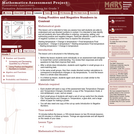Only Sharing Permitted
CC BY-NC-ND
Rating
0.0 stars

This lesson unit is intended to help teachers assess how well students are able to understand and use directed numbers in context. It is intended to help identify and aid students who have difficulties in ordering, comparing, adding, and subtracting positive and negative integers. Particular attention is paid to the use of negative numbers on number lines to explore the structures: starting temperature + change in temperature = final temperature final temperature Đ change in temperature = starting temperature final temperature Đ starting temperature = change in temperature.

Subject:
Mathematics
Numbers and Operations
Material Type:
Assessment
Lesson Plan
Provider:
Shell Center for Mathematical Education
Provider Set:
Mathematics Assessment Project (MAP)
04/26/2013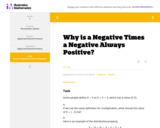Conditional Remix & Share Permitted
CC BY-NC-SA
Rating
0.0 stars

The purpose of this task is for students to understand the reason it makes sense for the product of two negative numbers to be positive. The idea is that if the properties of operations with which we are familiar when we do arithmetic with positive numbers are universal, then we have to define multiplication on signed numbers the way we do. The task only works through a single example, but the argument would work for any two negative numbers.

Subject:
Mathematics
Material Type:
Activity/Lab
Provider:
Illustrative Mathematics
Author:
Illustrative Mathematics